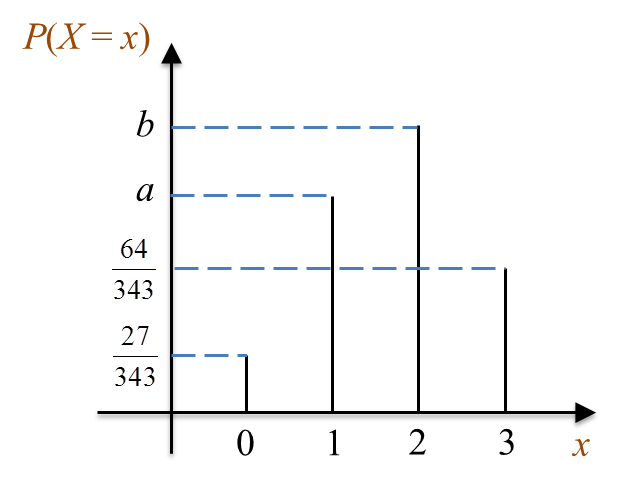# SPM Additional Mathematics 2017, Paper 1 (Question 23 – 25)

Question 23 (4 marks):
A set of data consists of 2, 3, 4, 5 and 6. Each number in the set is multiplied by m and added by n, where m and n are integers. It is given that the new mean is 17 and the new standard deviation is 4.242.
Find the value of m and of n.

Solution:

Question 24 (3 marks):
Diagram 9 shows the graph of binomial distribution X ~ B(3, p).Diagram 9

(a)
Express P(X = 0) + P(X > 2) in terms of a and b.
(b) Find the value of p.

Solution:
(a)
P(X = 0) + P(X = 1) + P(X = 2) + P(X = 3) = 1
P(X = 0) + a + b + P(X = 3) = 1
P(X = 0) + P(X = 3) = 1 – a – b
P(X = 0) + P(X > 2) = 1 – a – b

(b)

$\begin{array}{l}P\left(X=0\right)=\frac{27}{343}\\ {}^{3}{C}_{0}\left({p}^{0}\right){\left(1-p\right)}^{3}=\frac{27}{343}\\ 1×1×{\left(1-p\right)}^{3}={\left(\frac{3}{7}\right)}^{3}\\ 1-p=\frac{3}{7}\\ p=\frac{4}{7}\end{array}$

Question 25 (4 marks):
Diagram 10 shows a standard normal distribution graph.Diagram 10

The probability represented by the area of the shaded region is 0.2881.
(a) Find the value of h.
(b) X is a continuous random variable which is normally distributed with a mean, μ and a variance of 16.
Find the value of μ if the z-score of X = 58.8 is h.

Solution:
(a)
P(X < h) = 0.5 – 0.2881
P(X < h) = 0.2119
P(X < –0.8) = 0.2119
h = –0.8

(b)